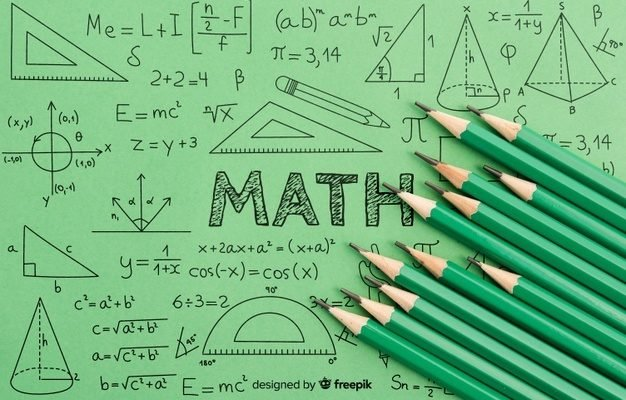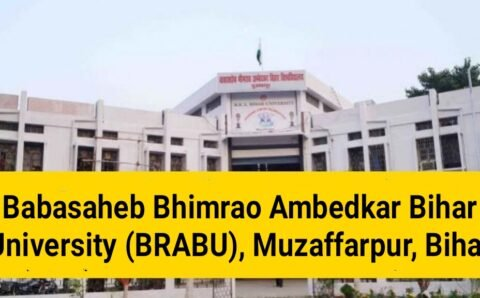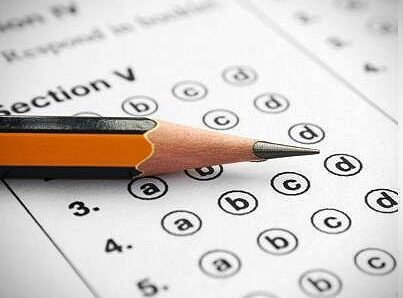# BIHAR UNIVERSITY (Muzaffarpur)MATHEMATICS Exam Important QuestionsBIHAR UNIVERSITY (Muzaffarpur) Exam Important Questions

(DAY 7)
15-03-2021
Graduation TDC Part 1 (2019-2022) Math/Physics/Chemistry/Zoology

Exam Important Questions
Graduation TDC Part 1 (2019-2022) Bihar University

PG 1st semester (2019-21) – Physics/Math – Important questions

Are you searching for BSc 1st year and PG 1st semester important questions in Math/Physics/Chemistry/Zoology ?

Well, you are in the right place. In this post, we are go to share some of the most important questions of Math/Physics/Chemistry (SCIENCE) for TDC Part 1 and PG 1st semester students. (B.R.A BIHAR UNIVERSITY)

TDC PART 1 MATHEMATICS (Hons.)
(Day 7)

Paper-2
TDC Part 1 (2019-22)

MATHEMATICS (Hons.) Paper- II

(Answer any six questions, selecting at least one from each group.)

Group-A

1. (a) In the curve r(1-cos 0) = 2a, prove p2 = a.r.

(b) State and prove Euler’s theorem on homogeneous functions of two Variables of degree n.

2 (a) State and prove Leibnitz’s theorem to find the nth derivative of a product of two functions of X.

(b) If Y y + Y-ym = 2x prove that

(x2 – 1) yn+2 + (2n + 1) xy,+1+ (n?M²) y, 0.

3. (a) Find the radius of curvature of a curve in Cartesian form. (b) Find the chord of curvature through the pole of the curve = a” cos Q.

Group B

1 (a) Find the area of the loop of the curve ay2 = x? (a – x)2 (b) Find the whole length of the loop of the curve 3 ay2 = x (x – 2)2

2(a) Find the volume of the right circulars cones of height h and base of Radius a.

(b) Find the area of the loop of the curve 32 = x (x – 1)2

Group C

1. (a) Find the equation of ellipse in standard form. (b) Find the equation of director circle to the conic =1+e cos 0

2. (a) Find the equation of the circle passing through the origin and cutting The circles x2 + y2 -4x + 6y + 10 = 0 and x2 + y2 + 12y + 6 = 0 Orthogonally.

(b) Find the equation of Radical acis of two circles.

3. (a) Find the equation of the tangent to the parabola y2 = 4ax at a point on it.

(b) Show that the radical axis of two circles is perpendicular to the line Joining their centres.

4. Find the condition for the circle X2 + y2 +28,*+2fy + = 0 And x2 + y2 +282x + 2/2y + 2 = 0 may cut each other orthogonally.

Group D

1. (a) Find the equation of the place in terms of the intercepts which it makes

On the axes.

(b) The length of two opposite edges of a tetrahedron are a, b; their shortest distance equal to d and the angle between them is 0. Prove That the volume is abd sin e.

2. (a) If I, m, n are the direction cosines of a line, then show that R+ m2 + 12 = 1.

(b) A line makes angles a, B, y with four diagonals of a cube. Prove that

Cosa + cos2B + cos?y + cos28 = 4/3.

3. (a) Find the volume of the tetrahedron whose co-ordinates of vertices are Given.

(b) Prove that every first degree equation in x, y, z represents a plane

THE END

Rn college Telegram group – Click here### स्नातक पार्ट-वन की परीक्षा के लिए क्वेश्चन बैंक बनाने में छूट रहे पसीने, हरेक विभाग को बनाना है 900 सवाल

बीआरए बिहार विश्वविद्यालय में स्नातक पार्ट-वन की परीक्षा के लिए क्वेश्चन बैंक तैयार करने में पीजी विभागों के पसीने छूट रहे हैं। हर विभाग को...### स्नातक पार्ट वन के परीक्षार्थियों को अगले माह मिलेगा मॉडल क्वेश्चन बैंक, परीक्षा के लिए मिल जाएगी अनुमति### BIHAR UNIVERSITY (Muzaffarpur)Zoology Exam Important Questions